# scikit-learn机器学习笔记——线性回归，岭回归1495-冯同学

, , ,

# 线性回归

h

(

w

)

=

w

0

+

w

1

x

1

+

w

2

x

2

+

h(w)=w_{0}+w_{1} x_{1}+w_{2} x_{2}+/cdots

h(w)=w0+w1x1+w2x2+

=

w

T

x

=w^{T} x

=wTx

w

,

x

w, x

w,x 为矩阵:

w

=

(

w

0

w

1

w

2

)

,

x

=

(

1

x

1

x

2

)

/mathbf{w}=/left(/begin{array}{c}w_{0} // w_{1} // w_{2}/end{array}/right), /mathbf{x}=/left(/begin{array}{c}1 // x_{1} // x_{2}/end{array}/right)

w=w0w1w2,x=1x1x2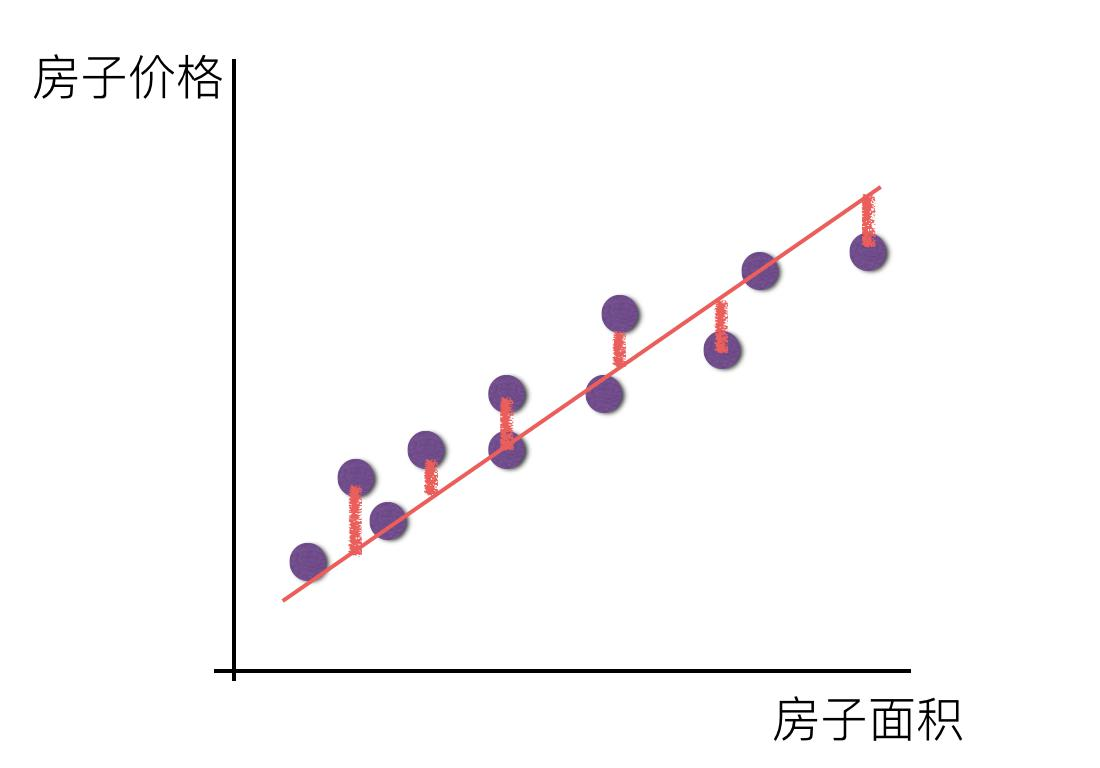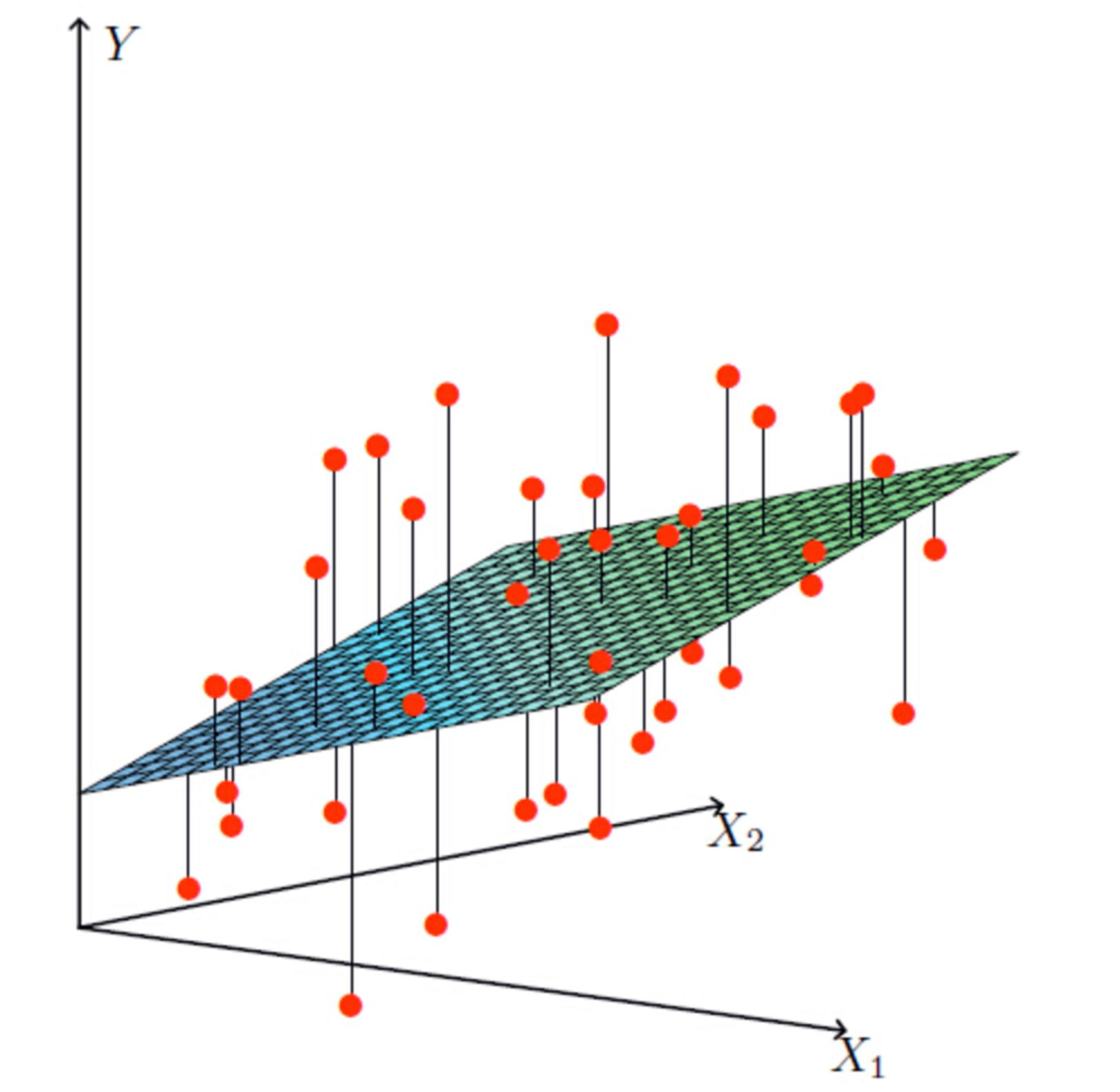## 损失函数

y

i

y_{i}

yi 为第i个训练样本的真实值

• h

w

(

x

i

)

h_{w}/left(x_{i}/right)

为第i个训练样本特征值组合预测函数
总损失定义:

J

(

θ

)

=

(

h

w

(

x

1

)

y

1

)

2

+

(

h

w

(

x

2

)

y

2

)

2

+

+

(

h

w

(

x

m

)

y

m

)

2

=

i

=

1

m

(

h

w

(

x

i

)

y

i

)

2

/begin{aligned} J(/theta) &=/left(h_{w}/left(x_{1}/right)-y_{1}/right)^{2}+/left(h_{w}/left(x_{2}/right)-y_{2}/right)^{2}+/cdots+/left(h_{w}/left(x_{m}/right)-y_{m}/right)^{2} // &=/sum_{i=1}^{m}/left(h_{w}/left(x_{i}/right)-y_{i}/right)^{2} /end{aligned}

### 最小二乘法直接求解

w

=

(

X

T

X

)

1

X

T

y

w=/left(X^{T} X/right)^{-1} X^{T} y

w=(XTX)1XTy

X

X

X 为特征值矩阵，

y

y

y 为目标值矩阵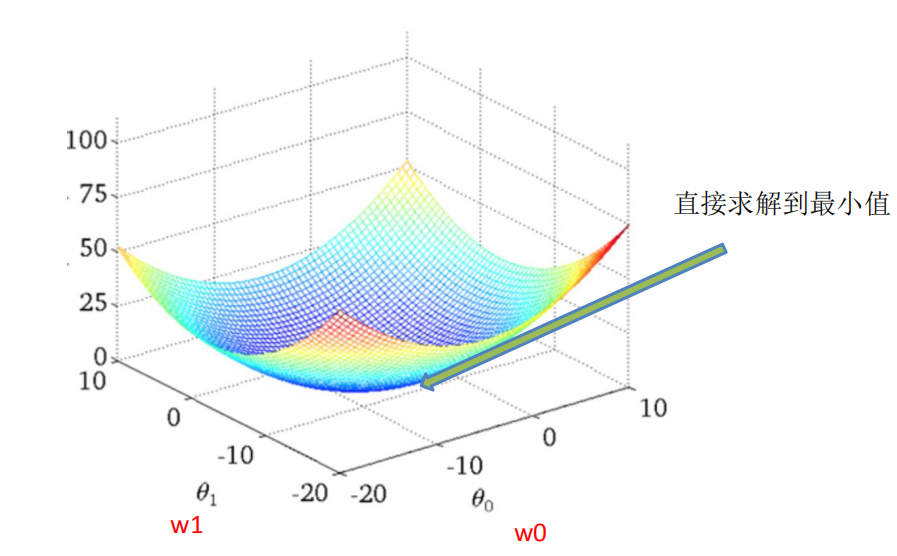### 最小二乘法梯度下降

w

1

:

=

w

1

α

cost

(

w

0

+

w

1

x

1

)

w

1

w

0

:

=

w

0

α

cost

(

w

0

+

w

1

x

1

)

w

1

/begin{array}{l} w_{1}:=-w 1-/alpha /frac{/partial /operatorname{cost}/left(w_{0}+w_{1} x_{1}/right)}{/partial w_{1}} // w_{0}:=-w_{0}-/alpha /frac{/partial /operatorname{cost}/left(w_{0}+w_{1} x_{1}/right)}{/partial w_{1}} /end{array}

w1:=w1αw1cost(w0+w1x1)w0:=w0αw1cost(w0+w1x1)

α

/alpha

α 为学习速率，需要手动指定,

cos

t

(

w

0

+

w

1

x

1

)

w

1

/frac{/partial /cos t/left(w_{0}+w 1 x 1/right)}{/partial w 1}

w1cost(w0+w1x1) 表示方向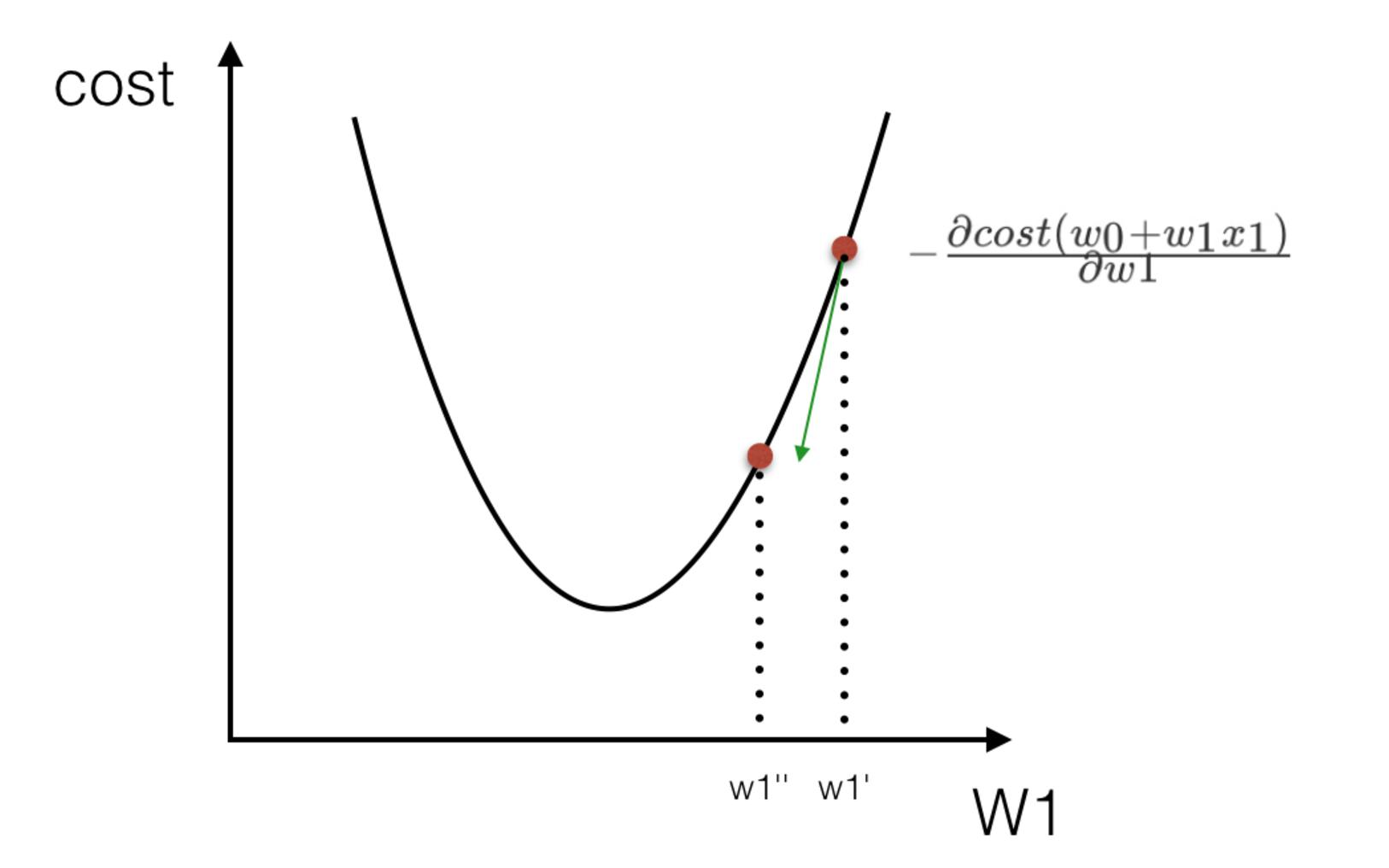## sklearn线性回归方程求解、梯度下降API

sklearn.linear_model.LinearRegression()
• 求解方程
• coef_：回归系数

sklearn.linear_model.SGDRegressor()
• 梯度下降
• coef_：回归系数

## 回归性能评估：

(均方误差(Mean Squared Error)MSE） 评价机制:

M

S

E

=

1

m

i

=

1

m

(

y

i

y

ˉ

)

2

M S E=/frac{1}{m} /sum_{i=1}^{m}/left(y^{i}-/bar{y}/right)^{2}

MSE=m1i=1m(yiyˉ)2

y

i

y^{i}

yi 为预测值，

y

ˉ

/bar{y}

yˉ为真实值。

## sklearn回归评估API

sklearn.metrics.mean_squared_error
mean_squared_error(y_true, y_pred)
• 均方误差回归损失
• y_true:真实值
• y_pred:预测值
• return:浮点数结果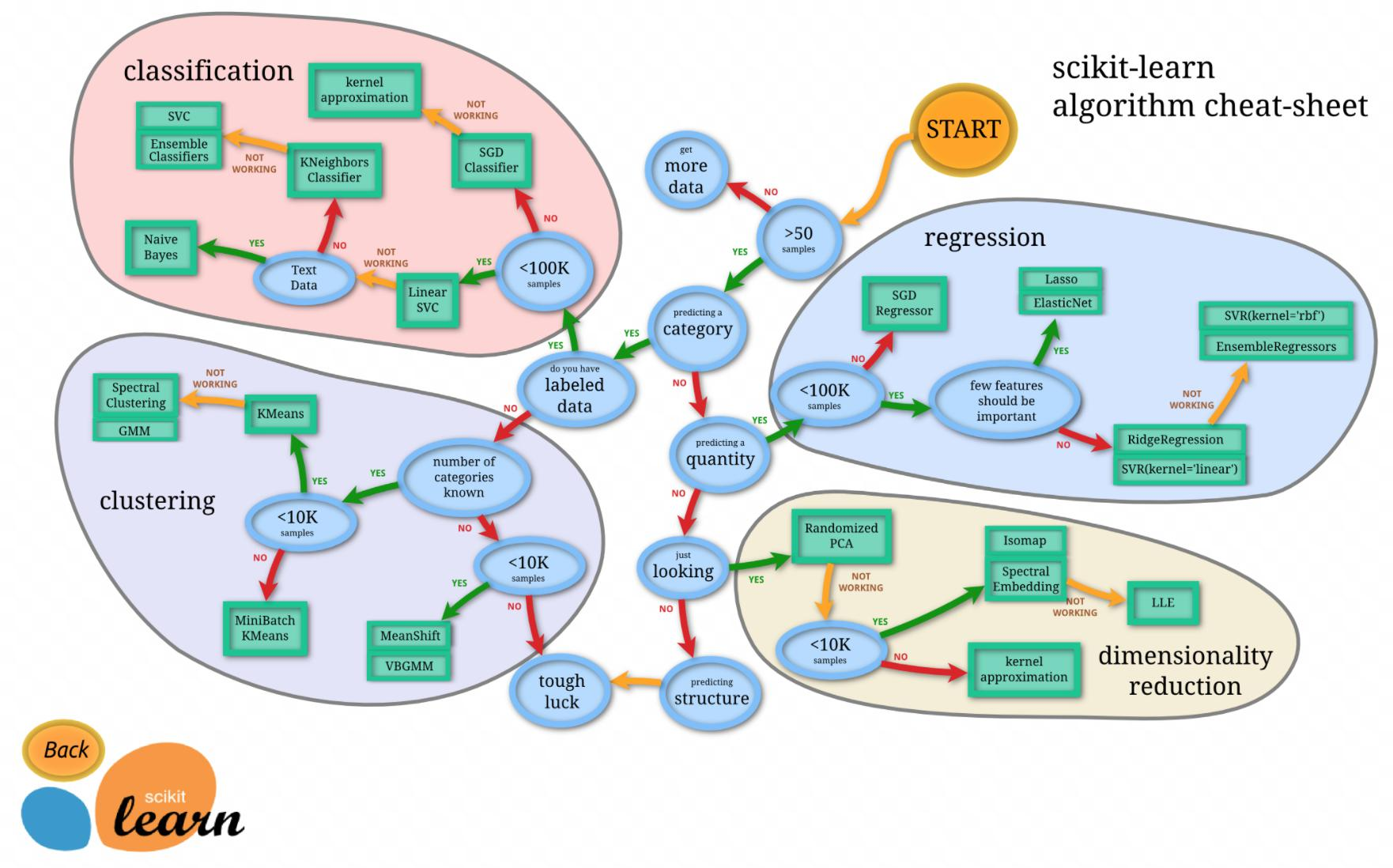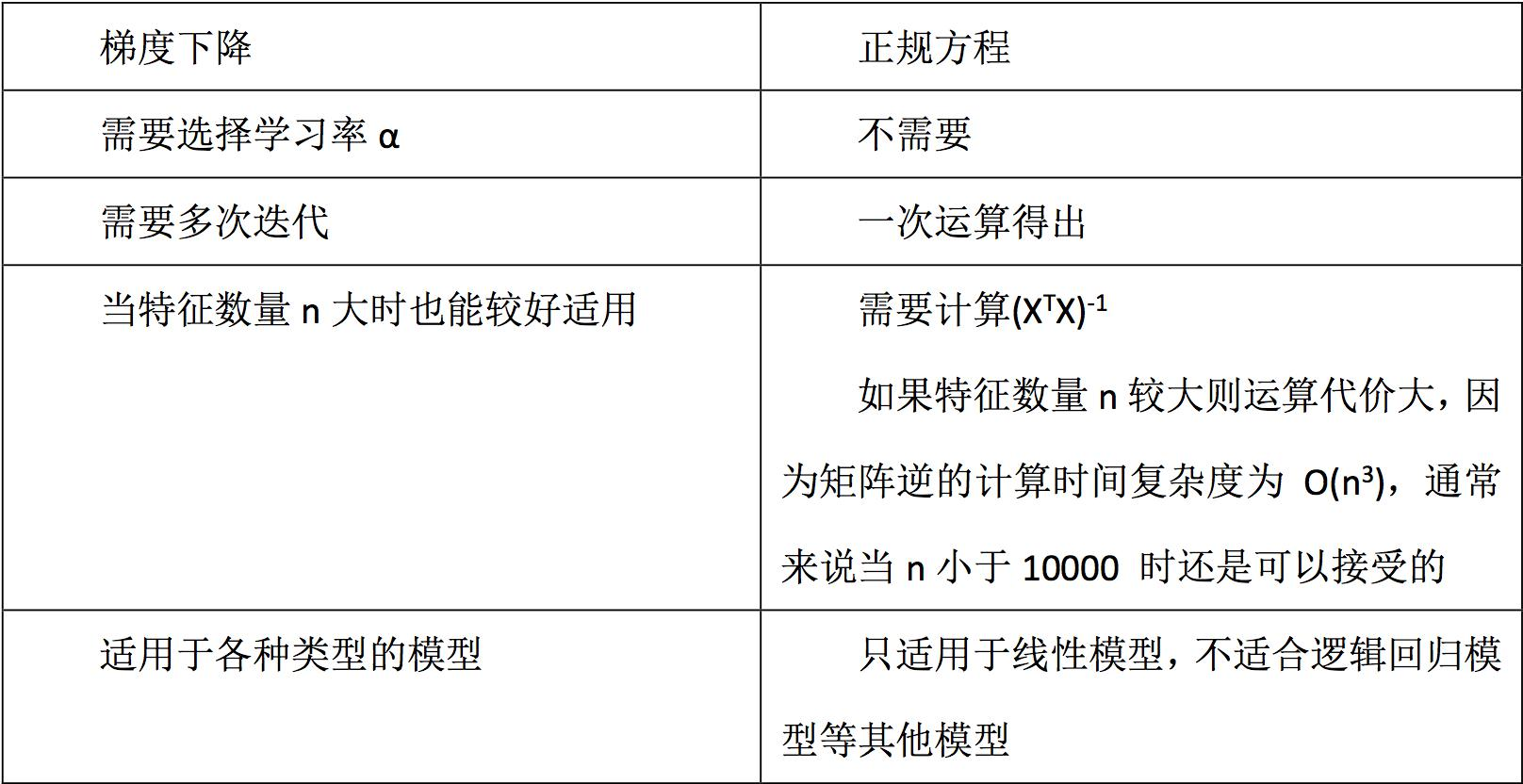## 线性回归实例

1、波士顿地区房价数据获取
2、波士顿地区房价数据分割
3、训练与测试数据标准化处理
4、使用最简单的线性回归模型LinearRegression和 梯度下降估计SGDRegressor对房价进行预测

from sklearn.linear_model import LinearRegression, SGDRegressor, Ridge
from sklearn.model_selection import train_test_split
from sklearn.preprocessing import StandardScaler
from sklearn.metrics import mean_squared_error
def linear_regression():
'''
线性回归预测波士顿房价
:return:
'''
# 读取数据
# 划分数据集
X_train, X_test, y_train, y_test = train_test_split(bh.data, bh.target, test_size=0.25)
# 标准化数据
std_X = StandardScaler()
X_train = std_X.fit_transform(X_train)
X_test = std_X.transform(X_test)

std_y = StandardScaler()
y_train = std_y.fit_transform(y_train.reshape(-1,1))
y_test = std_y.transform(y_test.reshape(-1,1))

# 实例化线性回归估计器
lr = LinearRegression()
lr.fit(X_train, y_train)
y_pred = std_y.inverse_transform(lr.predict(X_test))

#MSE
mse = mean_squared_error(y_pred=y_pred, y_true=std_y.inverse_transform(y_test))
print("线性回归求解方程的误差为:", mse)

# 实例化sgd
sgd = SGDRegressor()
sgd.fit(X_train, y_train)
y_pred1 = std_y.inverse_transform(sgd.predict(X_test))

#MSE
mse = mean_squared_error(y_pred=y_pred1, y_true=std_y.inverse_transform(y_test))
print("线性回归SGD的误差为:", mse)

# 实例化岭回归
rd = Ridge()
rd.fit(X_train, y_train)
y_pred2 = std_y.inverse_transform(rd.predict(X_test))

#MSE
mse = mean_squared_error(y_pred=y_pred2, y_true=std_y.inverse_transform(y_test))
print("岭回归的误差为:", mse)

if __name__ == '__main__':
linear_regression()



## 过拟合与欠拟合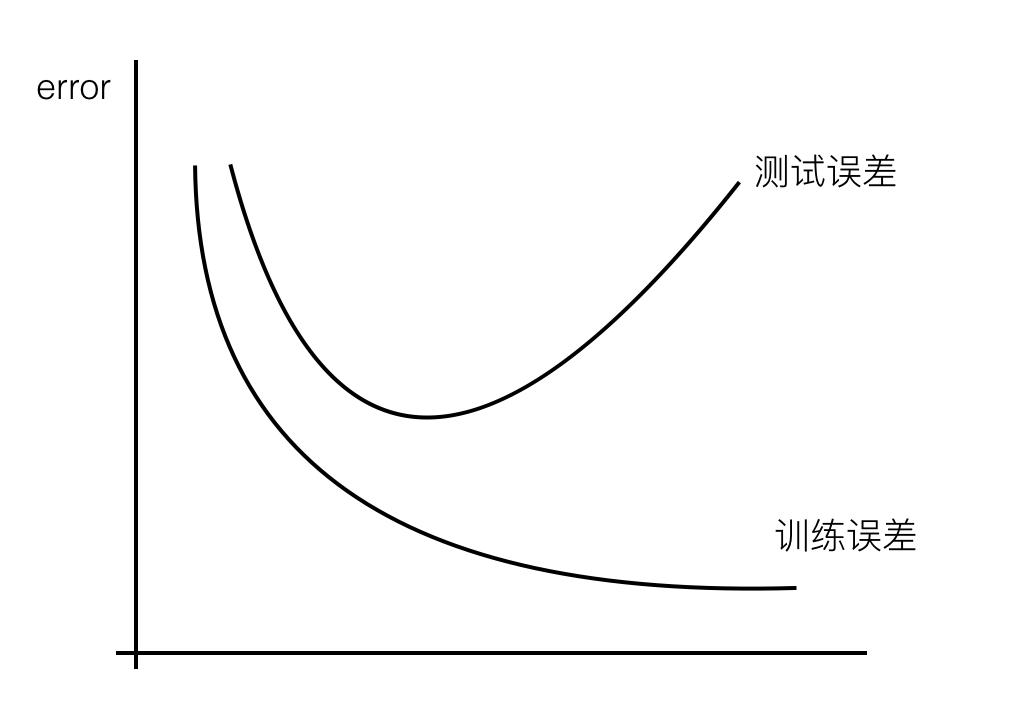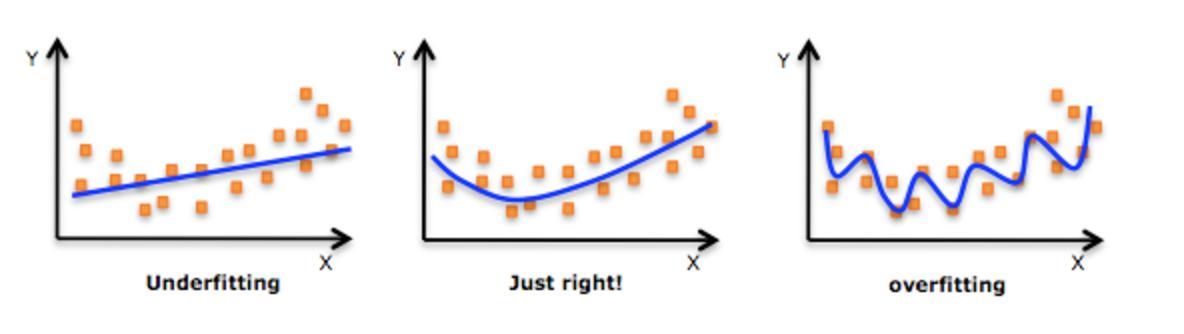### 降低过拟合和欠拟合的方法

1）添加其他特征项，有时候我们模型出现欠拟合，是因为特征项不够导致的，可以添加其他特征项来解决。例如，“组合”、“泛化”、“相关性”三类特征是特征添加的重要手段，无论在什么场景，都可以照葫芦画瓢，总会得到意想不到的效果。除上面的特征之外，“上下文特征”、“平台特征”等等，都可以作为特征添加的首选项。
2）添加多项式特征，这个在机器学习算法里面用的很普遍，例如将线性模型通过添加二次项或者三次项使模型泛化能力更强。例如，FM模型、FFM模型，其实就是线性模型，增加了二阶多项式，保证了模型一定的拟合程度。
3）减小正则化系数，前文中已经分析。

1）重新清洗数据，导致过拟合的一个原因也有可能是数据不纯导致的，如果出现了过拟合就需要我们重新清洗数据。
2）增加训练样本数量。
3）降低模型复杂程度。
4）增大正则项系数。
5）采用dropout方法，这个方法在神经网络里面很常用。dropout方法，通俗一点讲就是在训练的时候让神经元以一定的概率不工作。
6）early stoping。
7）减少迭代次数。
8）增大学习率。
9）添加噪声数据。
10）树结构中，可以对树进行剪枝。

### L2正则化

L2正则化：目标函数中增加所有权重w参数的平方之和, 逼迫所有w尽可能趋向零但不为零. 因为过拟合的时候, 拟合函数需要顾忌每一个点, 最终形成的拟合函数波动很大, 在某些很小的区间里, 函数值的变化很剧烈, 也就是某些w非常大. 为此, L2正则化的加入就惩罚了权重变大的趋势。

## 带有正则化的线性回归-Ridge

sklearn.linear_model.Ridge(alpha=1.0)
• 具有l2正则化的线性最小二乘法
• alpha:正则化力度
• coef_:回归系数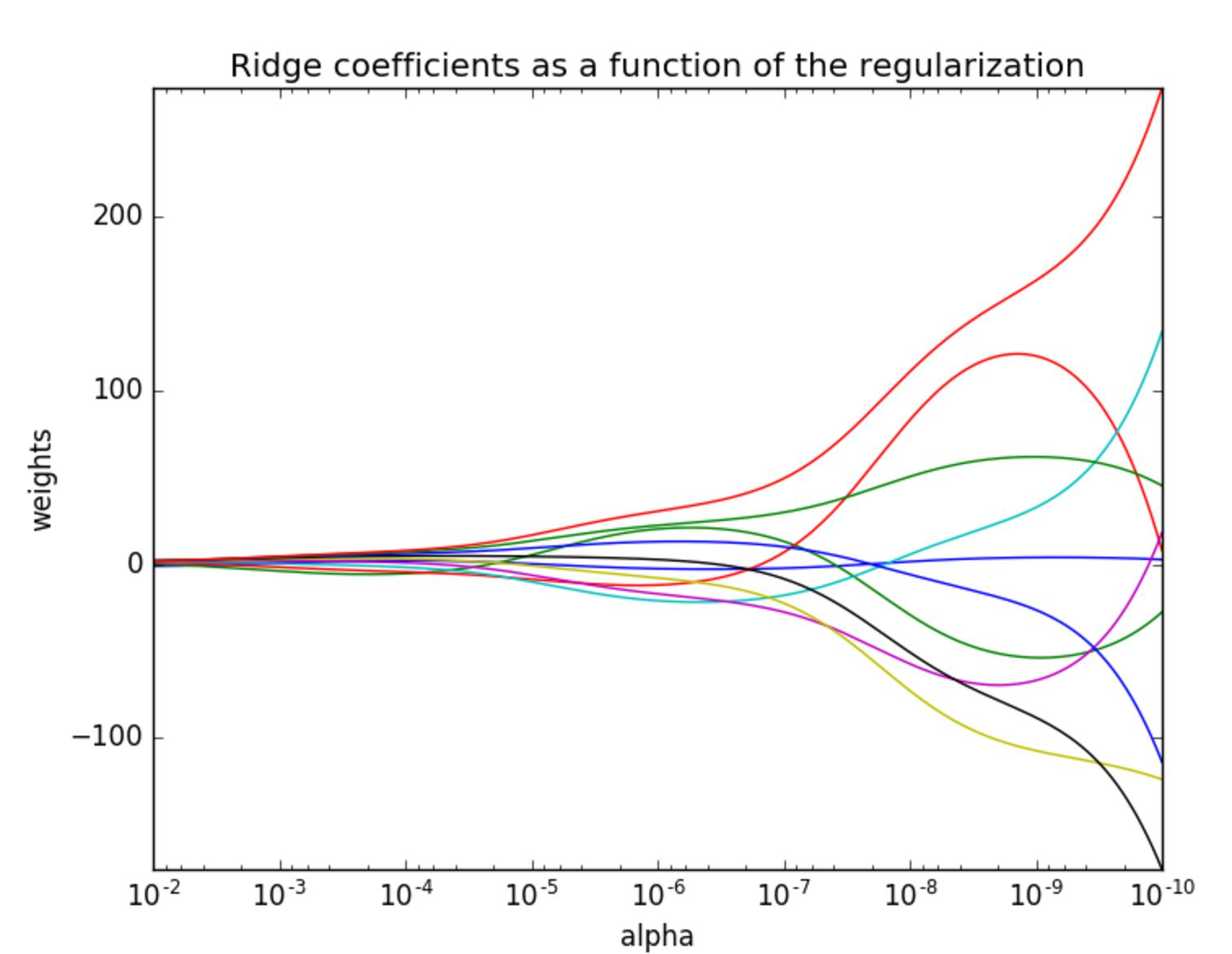### 评论 抢沙发### 觉得文章有用就打赏一下文章作者

#### 支付宝扫一扫打赏#### 微信扫一扫打赏Vieu3.3主题Q Q 登 录